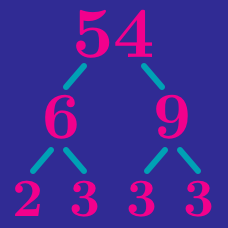Number Theory

# Prime Factorization and Divisors: Level 1 Challenges

$\Huge \frac{\color{#3D99F6}{50!}}{\color{#D61F06}{51}}$

What is the remainder of the division above?

Let $Y = n(n+1)$ for some positive integer $n$. Which of the following statements is true?

How many perfect squares are there between $101$ and $9999$?

Find the sum of the digits of $\large 2^{2999} × 5^{3002}$ when multiplied out.

Find the smallest positive integer which is both a perfect square and a perfect cube.

×